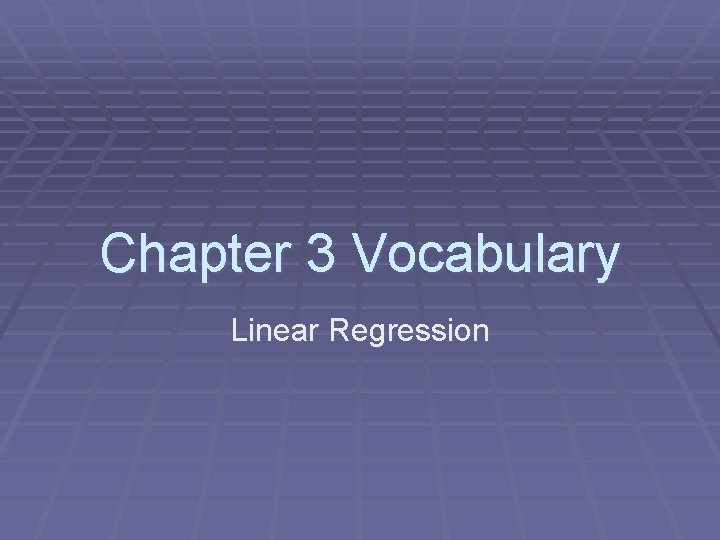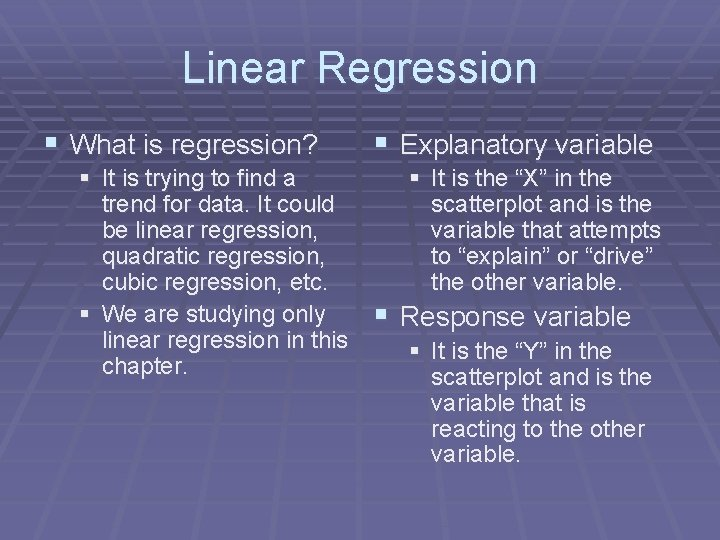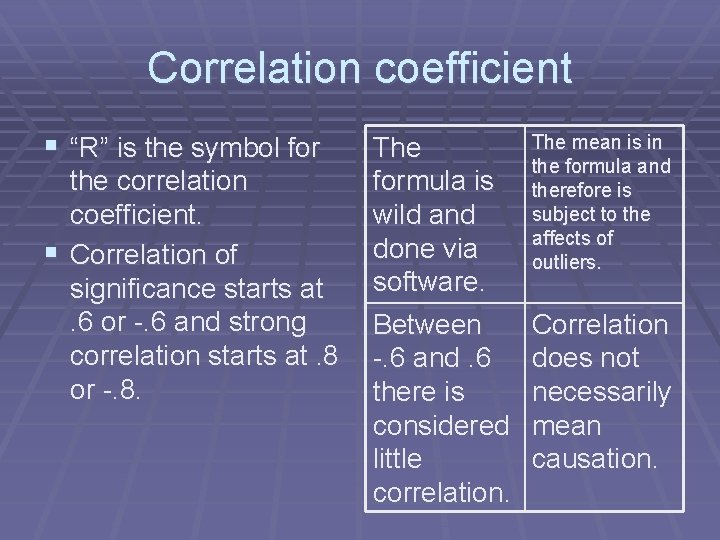# Chapter 3 Vocabulary Linear Regression Linear Regression What

• Slides: 6Chapter 3 Vocabulary Linear RegressionLinear Regression § What is regression? § It is trying to find a trend for data. It could be linear regression, quadratic regression, cubic regression, etc. § We are studying only linear regression in this chapter. § Explanatory variable § It is the “X” in the scatterplot and is the variable that attempts to “explain” or “drive” the other variable. § Response variable § It is the “Y” in the scatterplot and is the variable that is reacting to the other variable.Linear regression vocab § Least Squares Regression Line (LSRL) § This is a type of linear trend line. § There are other types of linear trend lines, such as the median-median line. This is the only linear trend line that we study in this course. § The name “least squares” comes from the fact that this line minimizes the sum of the squared residuals.Linear regression vocab § Residuals: this is the observed point – the predicted point. It is the vertical distance between the line and the actual point (show example) § Bivariate data: Most data with an x and a y value are graphed in a scatter plot. § Residual plot: shows the values of the residuals on a plot that is easy to read. Lack of a pattern shows that the line is a good fit for the data.Correlation coefficient § “R” is the symbol for the correlation coefficient. § Correlation of significance starts at. 6 or -. 6 and strong correlation starts at. 8 or -. 8. The formula is wild and done via software. Between -. 6 and. 6 there is considered little correlation. The mean is in the formula and therefore is subject to the affects of outliers. Correlation does not necessarily mean causation.Coefficient of determination § “R-squared” is the symbol. § Uses the same math as “R” but means something completely different. § It means “the percentage of the variability in y due to X. ” Outliers or influential points will affect greatly. You should memorize the catch phrase at left. The residual plot is a better measure of fit than is the value of R^2 Memorize the name for Rsquared.